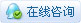899浏览

# AI实验——基于用户行为数据分析的智能家居（1）AI实验——基于用户行为数据分析的智能家居（1）
【项目源起】
随着科技的进步和人们生活水平的提高，人们对智能家居环境的要求也越来越高，如何满足人们家居智能化、个性化的要求是当前智能家居研究的目标。然而目前智能家居系统大多还处于一种单一的“机械式”自动化模式，其缺陷主要为“智能化程度低”。【项目简介】

本项目通过拿铁熊猫智能终端配合相关传感器采集用户数据，进行特征提取和模型训练，从而形成一个基于用户行为数据分析的个性化模型。在实际应用的过程中，当遇到数据进入模型可为用户自动做出是否打开空调的预测。
【项目原理】
基于用户行为数据分析的智能家居，在实施层面具体分为如下几个过程数据处理、模型训练、部署电器如图2所示，本文主要介绍数据处理这块的内容。（1）数据采集：利用一种装置，从系统外部采集数据并输入到系统内部的一个接口。数据采集技术广泛应用在各个领域。比如传感器、摄像头，麦克风，都是数据采集工具。除了上述方法外，我们还可以从从网络获取数据。
（2）数据预处理：由于采集到的数据可能有缺失、重复、错误或不统一，因此删除重复数据、补全缺失数据、校正错误数据和将数据处理成统一的标准。数据预处理的常用方法有数据清洗、数据编码、数据归一化或标准化等。数据预处理的过程就是将数据标准化的过程。
（3）数据可视化：是指将数据以恰当的方式呈现出来。数据可视化的作用是便于人们理解和使用数据，具有直观、生动和易于理解的优势。数据可视化的常用方法有折线图、直方图、柱状图、散点图等。
（4）数据分析：指运用恰当的分析方法和工具，对整理后的数据加以研究和总结，从中提取规律，最终形成结论。数据分析的常用方法有相关分析、对比分析、平均分析等。
【硬件器材】

 序号 名称 数量 1 拿铁熊猫智能终端 1 2 拿铁熊猫智能终端IO拓展板 1 3 摄像头 1 4 LED模块 1 5 蜂鸣器模块 1 6 USB转以太接口 2 7 网线 1 8 Gravity: 模拟环境光线传感器(Arduino兼容) 1 9 Gravity: DHT11温湿度传感器(Arduino兼容) 1 10 Gravity: 人体红外热释电运动传感器 1 11 Gravity: BMP388气压温度传感器 1 12 Gravity: CCS811空气质量传感器 1【采集数据】
我们使用传感器采集家庭各项数据，并将采集到的数据处理成表格，方便后续使用。采集到的数据可以保存为不同格式的文件，如csv文件、txt文件。其中，csv文件表示将数据表格存储为纯文本，每一行代表一条数据，每一条数据包含了一个值或由逗号分隔的多个值。例如，下图中，左侧为csv文件，右侧为原表格。
#导入相关库
``````import os.path

import csv

import time

import datetime

from datetime import timezone

import pytz

from pinpong.libs.dfrobot_bmp388 import BMP388

from pinpong.libs.dfrobot_ccs811 import CCS811

sampling_interval = 3# 采样间隔 3s

Board("leonardo").begin()

PIR_pin = Pin(Pin.D7, Pin.IN)       # 创建运动红外检测数字引脚实例

dht11 = DHT11(Pin(Pin.D6))        # 创建DHT11实例

bmp = BMP388()# 创建BMP实例

ccs811 = CCS811()# 创建ccs811实例

now = datetime.datetime.now()

date = now.strftime("%Y_%m_%d")

file_name = 'multi_sensors_data_logger_{}.csv'.format(date)

file_exists = os.path.isfile(file_name)

weekdays = {0:"周一",1:"周二",2:"周三",3:"周四",4:"周五",5:"周六",6:"周日"}

global index_

index_ = 1

def log_data(idx,ac_status):

with open(file_name, 'a', newline='') as csvfile:

field_names = ['index','business_day','PIR','working_time', 'light', 'temperature', 'humidity', 'pressure', 'co2', 'if_AC']

writer = csv.DictWriter(csvfile, fieldnames=field_names)

if not file_exists:

writer.writerow(

'humidity':'湿度','pressure':'气压','co2':'二氧化碳','if_AC':'是否开空调'})

weekday = weekdays[datetime.datetime.today().weekday()]# 工作日

now = datetime.datetime.now() # 当前的时间(世界时间)

now=now.replace(tzinfo=timezone.utc).astimezone(tz=pytz.timezone("Asia/Shanghai"))

if now.hour < 11 and now.hour >9:

period = '早晨'

elif now.hour<13 and now.hour>=11:

period = '中午'

elif now.hour<16 and now.hour>=13:

period = '下午'

elif now.hour<18 and now.hour>=16:

period = '傍晚'

else:

period = '夜间'

try:# DHT11 温湿度读取

temp = dht11.temp_c() #读取摄氏温度

humi = dht11.humidity() #读取湿度

except:

temp = None

humi = None

# BMP388 气压读取

pressure = bmp.pressure_pa() #读取气压

co2 = ccs811.CO2_PPM()

else:

co2 = None

if_AC = ac_status         # 空调状态

writer.writerow(

print("data logged:business_day:{} working_time:{} PIR:{} light:{} temperature:{} humidity:{} pressure:{} co2:{}".format(weekday,period,PIR,light,temp,humi,pressure,co2))

while True:  # 每隔一段时间进行一次环境数据采集

log_data(index_,0)

index_ += 1

time.sleep(sampling_interval)复制代码``````（1）在格物象“拿铁熊猫”窗口，右键点击 csv 文件，在弹出的界面中，点击“下载”，将 csv 文件下载到本地电脑；
（2）再打开格物象“PYTHON 编辑器”窗口，点击上传按钮，选中本地的csv文件，上传到“PYTHON 编辑器”中。

【制作数据集】【数据预处理】

（1）特征编码：在机器学习中，大多数算法都只能够处理数值型数据，不能处理文字，然而在现实中，许多数据集中都会有文字。比如下表中有很多是文字型数据。``````import pandas as pd

import numpy as np

import matplotlib.pyplot as plt

import time

from sklearn.model_selection import train_test_split

from sklearn.naive_bayes import GaussianNB, BernoulliNB, MultinomialNB

from sklearn import metrics

from sklearn.preprocessing import LabelEncoder, OrdinalEncoder, OneHotEncoder

import joblib

# 导入预先收集好的数据集

# 特征编码

#编码 1 是否开空调

ac_status = HAR_df.iloc[:]['是否开空调'].values # df.iloc[:,-1].values

labelencoder_ac = LabelEncoder()

ac_status = labelencoder_ac.fit_transform(ac_status)

HAR_df['是否开空调'] = ac_status# 特征编码
# 编码2 时间段
``````HAR_df['工作时段'] = HAR_df['工作时段'].replace(['早晨','中午','下午','傍晚'],'工作时间段')

HAR_df['工作时段'] = HAR_df['工作时段'].replace(['夜间'],'休息时间段')

period = HAR_df.iloc[:][['工作时段']].values

ordinalencoder = LabelEncoder()

period = ordinalencoder.fit_transform(period.ravel())

HAR_df['工作时段'] = period# 特征编码
``````# 编码3 工作日

HAR_df['工作日'] = HAR_df['工作日'].replace(['周一','周二','周三','周四','周五'],'工作日')

HAR_df['工作日'] = HAR_df['工作日'].replace(['周六','周日'],'假日')

daytype = HAR_df.iloc[:][['工作日']].values

ordinalencoder = LabelEncoder()

daytype = ordinalencoder.fit_transform(daytype.ravel())

HAR_df['工作日'] = daytype（2）数据清洗：采集到的原始数据，由于数据可能有缺失、重复、错误或不统一的情况，通常将这些数据进行数据清洗。在上述数据集中，可以删除多余的序号列。
``````# 删除多余的序号列

HAR_df = HAR_df.drop('序号',axis = 1)``````# 保存预处理后的数据为csv文件

HAR_df.to_csv("HAR_dataset_REAL_preprocessed.csv",index = False)复制代码``````

【数据可视化】

#环境数据可视化与数据分析
``````# 导入相关库

import pandas as pd

import numpy as np

import matplotlib.pyplot as plt

import time

from sklearn.model_selection import train_test_split

from sklearn.naive_bayes import GaussianNB, BernoulliNB, MultinomialNB

from sklearn import metrics

from sklearn.preprocessing import LabelEncoder, OrdinalEncoder, OneHotEncoder

import joblib

# 导入预处理后的数据集

# 新建一个dataframe，保留温度，二氧化碳和是否开空调三个特征

HAR_df_temp_co2 = HAR_df[['温度','二氧化碳','是否开空调']]``````# 绘制散点图

figsize = (20,6)

alpha = 0.6

marker_size = 50

fig, axes = plt.subplots(1, 1)

fig.suptitle("Will temperature and CO2 concentration affect the opening of the air conditioner?",fontsize=16)

HAR_df_temp_co2.loc[HAR_df_temp_co2['是否开空调'] == 1].plot.scatter(x="二氧化碳",y="温度",c='b',marker='o',alpha = alpha,s=marker_size,ax=axes, figsize=figsize,label = 'AC_ON')

HAR_df_temp_co2.loc[HAR_df_temp_co2['是否开空调'] == 0].plot.scatter(x="二氧化碳",y="温度",c='r',marker='o',alpha = alpha,s=marker_size,ax=axes, figsize=figsize,label = 'AC_OFF')

axes.set_xlabel("CO2 Concentration")

axes.set_ylabel("Temperature")复制代码``````

``````# 新建一个dataframe，保留工作日，温度，二氧化碳和是否开空调三个特征

HAR_df_busday_temp_co2 = HAR_df[['工作日','温度','二氧化碳','是否开空调']]``````# 绘制散点图

ig, axes = plt.subplots(1, 2)

fig.suptitle("Will temperature and CO2 concentration affect the opening of the air conditioner?",fontsize=16)

axes.title.set_text("weekend")

for idx,axis in enumerate(axes):

HAR_df_busday_temp_co2.loc[(HAR_df_busday_temp_co2['工作日'] == idx) & (HAR_df_busday_temp_co2['是否开空调'] == 1)].plot.scatter(x="二氧化碳",y="温度",c='b',marker='o',alpha = alpha,s=marker_size,ax=axis, figsize=figsize,label = 'AC_ON')

HAR_df_busday_temp_co2.loc[(HAR_df_busday_temp_co2['工作日'] == idx) & (HAR_df_busday_temp_co2['是否开空调'] == 0)].plot.scatter(x="二氧化碳",y="温度",c='r',marker='o',alpha = alpha,s=marker_size,ax=axis, figsize=figsize,label = 'AC_OFF')

axis.set_xlabel("CO2 Concentration")

axis.set_ylabel("Temperature")复制代码````````````# 新建两个dataframe，保留工作日，工作时段，温度，二氧化碳和是否开空调三个特征。

HAR_df_busday_workinghour_temp_co2 = HAR_df[['工作日','工作时段','温度','二氧化碳','是否开空调']].loc[HAR_df['工作时段'] == 1]

HAR_df_busday_nonworkinghour_temp_co2 = HAR_df[['工作日','工作时段','温度','二氧化碳','是否开空调']].loc[HAR_df['工作时段'] == 0]``````# 绘制散点图

figsize = (20,6)

alpha = 0.6

marker_size = 50

wokring_hour = ["non wokring hour","wokring hour"]

for idx,wokring_hour in enumerate(wokring_hour):

fig, axes = plt.subplots(1, 2)

fig.suptitle("period: {}".format(wokring_hour),fontsize=16)

axes.title.set_text("weekend")

HAR_df_busday_workinghour_temp_co2.loc[(HAR_df_busday_workinghour_temp_co2['工作日'] == idx) & (HAR_df_busday_workinghour_temp_co2['是否开空调'] == 1)].plot.scatter(x="二氧化碳",y="温度",c='b',marker='o',alpha = alpha,s=marker_size,ax=axes, figsize=figsize,label = 'AC_ON')

HAR_df_busday_workinghour_temp_co2.loc[(HAR_df_busday_workinghour_temp_co2['工作日'] == idx) & (HAR_df_busday_workinghour_temp_co2['是否开空调'] == 0)].plot.scatter(x="二氧化碳",y="温度",c='r',marker='o',alpha = alpha,s=marker_size,ax=axes, figsize=figsize,label = 'AC_OFF')

HAR_df_busday_nonworkinghour_temp_co2.loc[(HAR_df_busday_nonworkinghour_temp_co2['工作日'] == idx) & (HAR_df_busday_nonworkinghour_temp_co2['是否开空调'] == 1)].plot.scatter(x="二氧化碳",y="温度",c='b',marker='o',alpha = alpha,s=marker_size,ax=axes, figsize=figsize,label = 'AC_ON')

HAR_df_busday_nonworkinghour_temp_co2.loc[(HAR_df_busday_nonworkinghour_temp_co2['工作日'] == idx) & (HAR_df_busday_nonworkinghour_temp_co2['是否开空调'] == 0)].plot.scatter(x="二氧化碳",y="温度",c='r',marker='o',alpha = alpha,s=marker_size,ax=axes, figsize=figsize,label = 'AC_OFF')

axes.set_xlabel("CO2 Concentration")

axes.set_ylabel("Temperature")

axes.set_xlabel("CO2 Concentration")

axes.set_ylabel("Temperature")  复制代码``````### 评分DFROBOT317 + 5 + 5 + 2 --------
 您需要登录后才可以回帖 登录 | 立即注册 本版积分规则 回帖并转播 回帖后跳转到最后一页